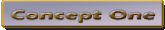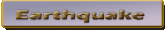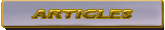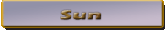Introduction to SunTerratan X = CE / (SA+AB+BE)

Solving for X which is half the angle of the opening of SunTerra beam.

Tan X = 4000 / (435000+93000000+4000) = 0.0000428

Then X = 1/407 degree

& Y = 2 X = 1/203.5

AD = tan X x SA

AD = 0.0000428 x 435000 = 18.61 ( in miles )

SunTerra Belt is referred to the total surface area around the Sun composed of 18.61 miles in radius circles of SunTerra Discs that provide light to the Earth as moves in its orbit around the Sun.

A presentation of SunTerra for the Solar System by scale down factor of 1/6000000000

Following calculations are in metric system.

Sun

435000 x 1.61 / 6000000000 = 0.00011 km

0.00011 x 100000 = 11 cm

Earth

4000 x 1.61 / 6000000000 = 0.000001 km

0.000001 x 1000000 = 1 mm

Distance

93000000 x 1.61 / 6000000000 = 0.0249 km

0.0249 x 1000 = 24.9 m

SunTerra Laws Coming Soon.

Email: conceptonesunterra@gmail.com